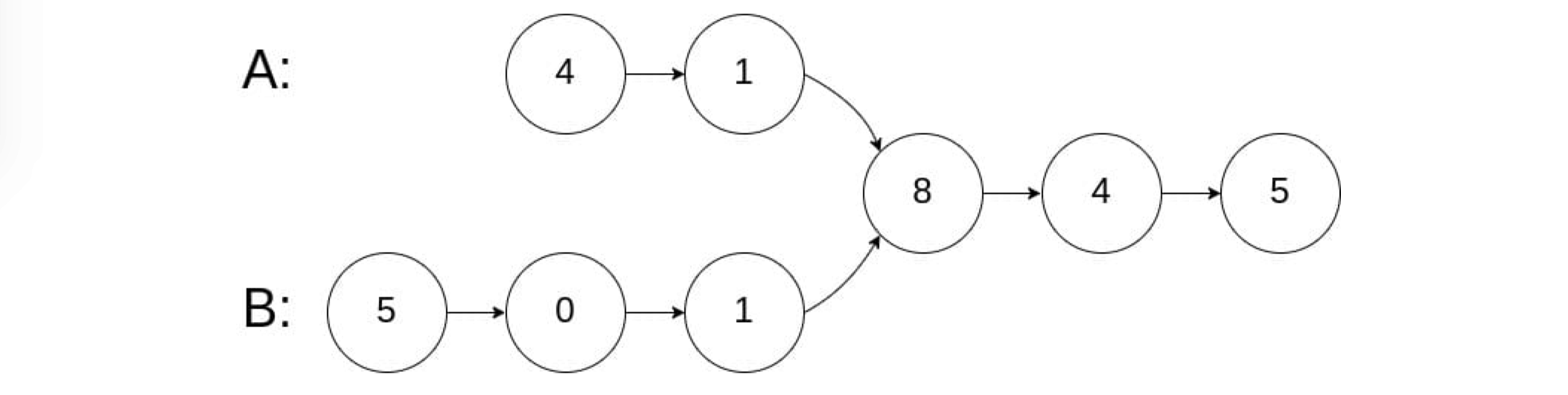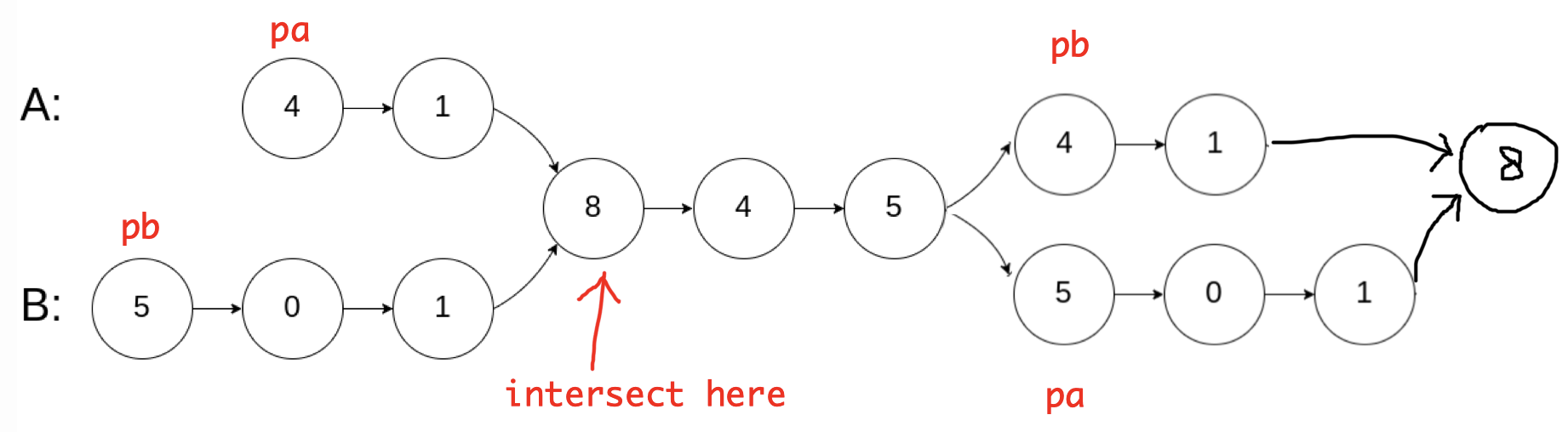# 160. Intersection of Two Linked Lists

Reference: LeetCode EPI 7.4
Difficulty: EasyTwo Pointers

## Problem

Write a program to find the node at which the intersection of two singly linked lists begins.

Note:

• If the two linked lists have no intersection at all, return null.
• The linked lists must retain their original structure after the function returns.
• You may assume there are no cycles anywhere in the entire linked structure.
• Your code should preferably run in $O(n)$ time and use only $O(1)$ memory.

Example:## Analysis

### Brute-Force

For each node in list $A$, traverse the entire list $B$ and check if any node in list
$B$ coincides with the node in list $A$.

Time: $O(mn)$
Space: $O(1)$

### Hash Table

Traverse list $A$ and store the address to each node in a hash set.

Time: $O(m + n)$
Space: $O(m)$ or $O(n)$

### Two Pointers

Two pointers pa and pb respectively start from list $A$ and list $B$.

• If pa reaches the end, it then points to the head of list $B$.
• If pb reaches the end, it then points to the head of list $A$.

We only change pa and pb in that way once.

• If they coincide later, return true.
• If one of them reaches null, return false.
• Or we can implement without the once logic. See details below.Code:

Time: $O(m + n)$
Space: $O(1)$s

### Length Difference

Get the length of each list and calculate the difference. According to this difference, one list moves in advance by steps. Then move in tandem and they will reach the intersection node if it exists; otherwise, one of them may reach the end and the program returns null.

Time: $O(m + n)$
Space: $O(1)$

CommentJunhao Wang
a software engineering cat